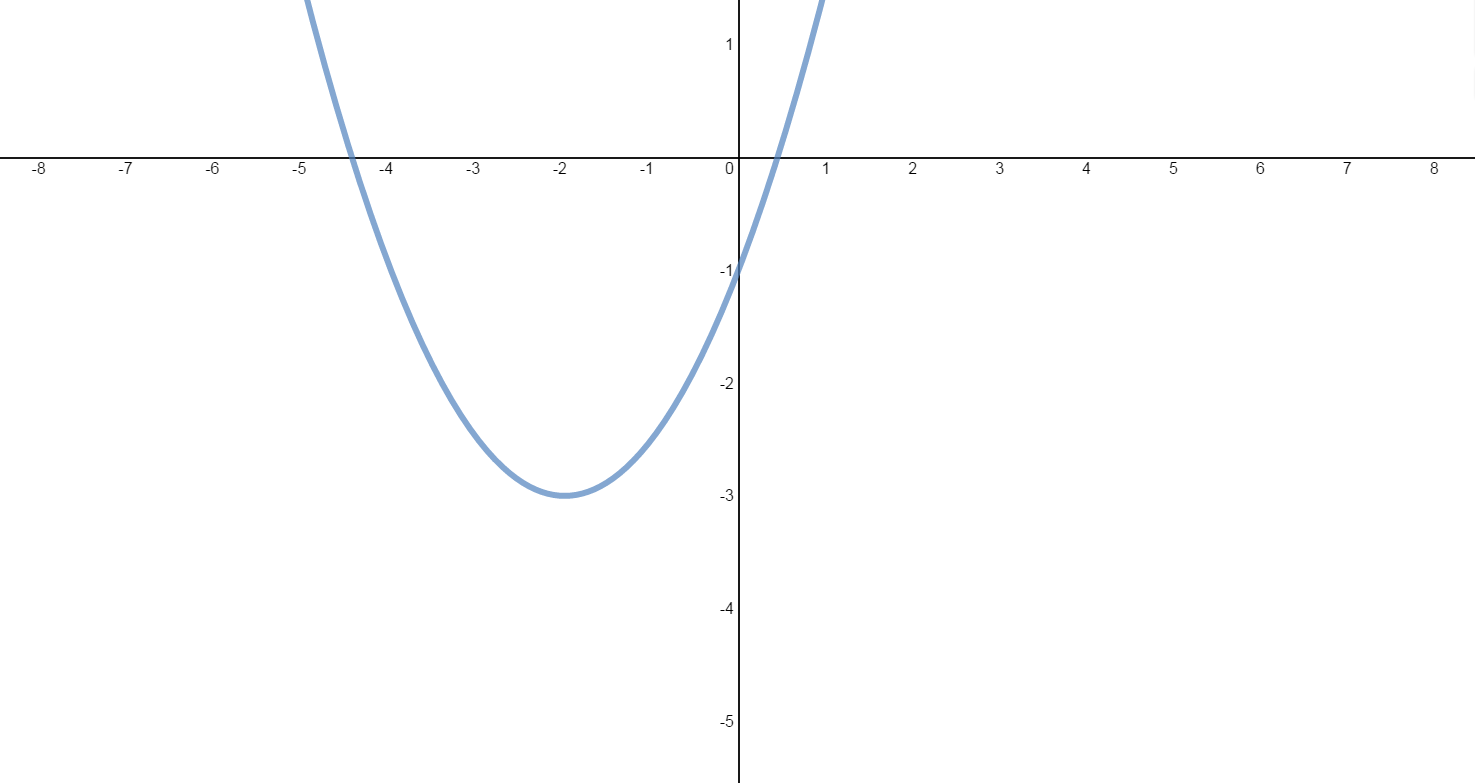# Slope and equation of tangent line#### Everything You Need in One Place

Homework problems? Exam preparation? Trying to grasp a concept or just brushing up the basics? Our extensive help & practice library have got you covered.#### Learn and Practice With Ease

Our proven video lessons ease you through problems quickly, and you get tonnes of friendly practice on questions that trip students up on tests and finals.#### Instant and Unlimited Help

Our personalized learning platform enables you to instantly find the exact walkthrough to your specific type of question. Activate unlimited help now!##### Intros
###### Lessons
1. Connecting: Derivative & Slope & Equation of Tangent Line
Exercise: The graph of the quadratic function $f\left( x \right) = \frac{1}{2}{x^2} + 2x - 1$ is shown below.2. Find and interpret $f'\left( x \right)$.
3. Find the slope of the tangent line at:
i) $x = - 1$
ii) $x = 2$
iii) $x = - 7$
iv) $x = - 4$
v) $x = - 2$
4. Find an equation of the tangent line at:
i) $x = 2$
ii) $x = - 4$
iii) $x = - 2$
##### Examples
###### Lessons
1. Determining Equations of the Tangent Line and Normal Line
Consider the function: $f(x)=\frac{x}{32}(\sqrt{x}+{^3}\sqrt{x})$
1. Determine an equation of the tangent line to the curve at $x=64$.
2. Determine an equation of the normal line to the curve at $x=64$.
2. Locating Horizontal Tangent Lines
1. Find the points on the graph of $f(x)=2x^3-3x^2-12x+8$ where the tangent is horizontal.
2. Find the vertex of each quadratic function:
$f(x)=2x^2-12x+10$
$g(x)=-3x^2-60x-50$
3. Locating Tangent Lines Parallel to a Linear Function
Consider the Cubic function: $f(x)=x^3-3x^2+3x$
i) Find the points on the curve where the tangent lines are parallel to the line $12x-y-9=0$.
ii) Determine the equations of these tangent lines.
1. Determining Lines Passing Through a Point and Tangent to a Function
Consider the quadratic function: $f(x)=x^2-x-2$
1. Draw two lines through the point (3, -5) that are tangent to the parabola.
2. Find the points where these tangent lines intersect the parabola.
3. Determine the equations of both tangent lines.
2. Locating Lines Simultaneously Tangent to 2 Curves
$f(x)=x^2$
$g(x)=\frac{1}{4}x^2+3$
Point-Slope Form of a line with slope m through a point $(x_1,y_1): m=\frac{y-y_1}{x-x_1}$